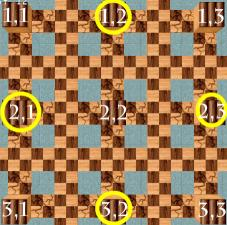World 10 - Level 8 Mathematical Wonderland Answer to each puzzle: 1. Since five plus one is six, and six squared is 36, the answer is five. Count from left to right and push the fifth box into the water. The fake wall contains a coin, all others contain Chompers (bad news!). 2. Prime numbers are 2,3,5, and 7 (not 1... it only has one divisor, itself!). Push boxes into each of these paths (counted from above) to get four more coins. 3. Two numbers (!) satisfy this problem, two and three. Build those two bridges. And now the final problem:There are five coins left, but one is close to the starting position, so we know that there are four in the array. The first clue discounts (1,1), (2,2) and (3,3) - row and column number can't be equal. The second clue discounts (1,3) and (3,1), since they cannot add up to four - the number of remaining coins. Hence the four coins are in the four circled positions. Finally, time your steps with the cannons - wait until they fire and quickly step on the button and push the boxes into the water... wait too long and the cannons will destroy your box!